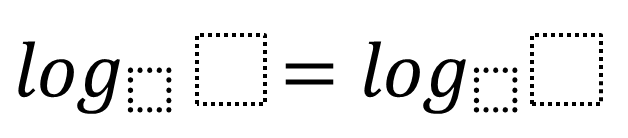# Logarithms

Directions: Using the digits 1 to 9 at most one time each, place a digit in each box to create two different solutions to the problem:### Hint

2 to what power equals 64?

Base = 4/2 Answer = 6/1
Base = 8/4 Answer = 6/1
Base = 4/1 Answer = 9/3
Base = 4/1 Answer = 6/2
Base = 8/2 Answer = 9/3
Base = 8/2 Answer = 3/1
Base = 8/1 Answer = 4/2
Base = 8/1 Answer = 6/3

Source: Noel Chang

## Period of Trig Function 3

Directions: Using the digits 0 to 9, at most one time, fill in the blanks …